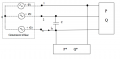# Three phase system question

#### Manuel Faria

Joined May 5, 2016
3
I have the following circuit. Three phase generator, direct sequenceThe goal is to calculate i3.

I know that e3(t)=200sqrt2cos(wt)
P=10kW Q=10kVAr
P*=10kW Q*=-5KVAr

What I'm doing:
e1(t)=200sqrt2cos(wt-120)
e2(t)=200sqrt2cos(wt-240)

i3'=V2(phase)/Zc
i3''=S/(sqrt(3)*VL)
i3'''=S*/(sqrt(3)*VL)
My doubt is in i3'' and i3'''
i3'=200sqrt2e^j(-240º)/j=283e^j(30)
i3''=10000sqrt(2)e^j(45)/sqrt(3)sqrt(3)200sqrt(2)e^j(30)=1,6e^j(15)
i3'''=11180e^j(27)/sqrt(3)200sqrt(2)e^j(30)=22,8e^j(-3)

Is this procedure correct? Thanks

#### WBahn

Joined Mar 31, 2012
28,490
I don't see anything that indicates whether or not the 3-phase load is balanced. Is it?

#### Manuel Faria

Joined May 5, 2016
3
It just says that the network three phase network is powered by three symmetric direct voltages.

#### WBahn

Joined Mar 31, 2012
28,490
Then you don't have much of a realistic choice but to assume that it is balance, but you should be sure to make a note that you are making that assumption.

•Manuel Faria

#### Manuel Faria

Joined May 5, 2016
3
So the way I'm thinking it is correct?

#### WBahn

Joined Mar 31, 2012
28,490
Possibly. I'm just not willing to wade through your work unless you go back and track your units through the work properly.